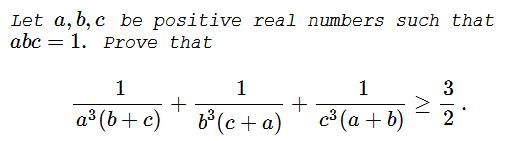# Problem 2, the 36th IMO (1995)

### Problem### Solution 1

Let's make a substitution $a=\displaystyle\frac{1}{x},\,$ $b=\displaystyle\frac{1}{y},\,$ $c=\displaystyle\frac{1}{z}.\,$ Still, $xyz=1\,$ and the inequality becomes

$\displaystyle \frac{x^3yz}{y+z}+\frac{y^3zx}{z+x}+\frac{z^3xy}{x+y}\overset{xyz=1}{=}\frac{x^2}{y+z}+\frac{y^2}{z+x}+\frac{z^2}{x+y}\ge\frac{3}{2}.$

If $S=\displaystyle\frac{x^2}{y+z}+\frac{y^2}{z+x}+\frac{z^2}{x+y}\,$ then, by the Cauchy-Schwarz inequality,

$[(y+z)+(z+x)+(x+y)]\cdot S\ge (x+y+z)^2,$

implying $\displaystyle S\ge\frac{x+y+z}{2}\ge\frac{3\sqrt{xyz}}{2}=\frac{3}{2},\,$ with equality when $x=y=z=1,\,$ i.e., for $a=b=c=1.$

### Solution 2

Starting with the same substitution as in Solition 1, we prove

$\displaystyle\frac{x^2}{y+z}+\frac{y^2}{z+x}+\frac{z^2}{x+y}\ge\frac{3}{2}$

simply with Bergström's inequality, followed by Cauchy-Schwarz inequality inequality as above.

### Solution 3

Consider point $\displaystyle P=\left(\frac{1}{(a^3(b+c))^{1/2}},\frac{1}{(b^3(c+a))^{1/2}},\frac{1}{(c^3(a+b))^{1/2}}\right)\in\mathbb{R}^3\,$ and plane $\mathcal{P} :\,(ab+ac)^{1/2}x+(bc+ba)^{1/2}y+(ca+cb)^{1/2}z=0.\,$ Then $O(0,0,0)\in\mathcal{P}.\,$ Denote by $D(P,O)\,$ the distance from $P\,$ to $O\,$ and by $D(P,\mathcal{P})\,$ the distance from $P\,$ to $\mathcal{P},\,$ respectively. It's easy to see that

$\displaystyle D^2(P,O)=\frac{1}{a^3(b+c)}+\frac{1}{b^3(c+a)}+\frac{1}{c^3(a+b)}$

and, by using the distance formula from a point to a plane (and omitting some of the tedious algebra here), we get

\displaystyle\begin{align} D^2(P,\mathcal{P})&=\frac{\displaystyle\left(\frac{1}{a}+\frac{1}{b}+\frac{1}{c}\right)^2}{2(ab+bc+ca)}\\ &=\frac{ab+bc+ca}{2}\\ &\ge\frac{3(ab\cdot bc\cdot ca)^{1/3}}{2}=\frac{3}{2}. \end{align}

Since $D^2(P,O)\ge D^2(P,\mathcal{P}),\,$ the result follows.

### Solution 4

First, let $p=a+b+c,\,$ $q=ab+bc+ca,\,$ $r=abc.\,$ By the AM-GM inequality $q\ge 3\,(1).\,$ Further, $q^2\ge 3pr=3p,\,$ so that $3p\le q^2\,(2).$

Rewrite the given inequality as

$\displaystyle E=\sum_{cycl}\frac{b^3c^3}{b+c}\ge\frac{3}{2}.$

\displaystyle\begin{align} 3\cdot E\cdot 2p &= E\cdot ((b+c)+(c+a)+(a+b))(1+1+1)\\ &\overset{Hölder}{\ge}(bc+ca+ab)^3, \end{align}

implying $\displaystyle E\ge\frac{q^3}{3(2p)}.\,$ With (2), we get $\displaystyle E\ge\frac{q^3}{2q^2}=\frac{q}{2}.\,$ Now, via (1),

$\displaystyle E=\frac{q}{2}\ge\frac{3}{2}.$

Equality for $a=b=c=1.$

### Acknowledgment

I came up to considering this problem on receiving Sidney Kung's communication on applications of the Cauchy-Schwarz inequality. Solution 3 is a part of this communication. Solution 1 is from The IMO Compendium by Dusan Djukic et al.; Solution 4 is by Imad Zak.

Aditional solutions are available online in an article Several proofs and generalizations of a fractional inequality with constraints by Fuhua Wei and Shanhe Wu.